Plücker Coordinates for the Rest of Us - Part 4 - Applications by Lionel Brits (15 November 2001) Return to The Archives
 Basic Properties of Plücker CoordinatesNot all Plücker coordinates correspond to real lines in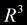. In the first representation, a necessary condition for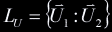to be real is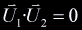,given that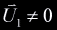(a line must have a direction), assuming that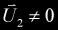(lines not passing through the origin; recall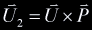). Since lines passing through the origin are just as real as other lines, this test only rules out non-real lines, but doesn't confirm that a line is real. In the second representation, a line is real when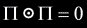Furthermore, two Plücker coordinatesand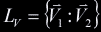describe the same line if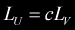for some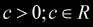and describe equal but oppositely directed lines for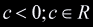. Also linesandare perpendicular whenand parallel when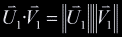, although these two points are simple consequences from the geometry upon which Plücker coordinates are based.

 Plane-Line IntersectionRecall that the equation that defines a plane with normaland distance from the origin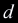, the set of points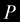such that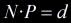.Given a planeand a line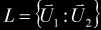, the homogeneous coordinate for the intersection of the two is given by: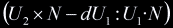which in Cartesian coordinates is equivalent to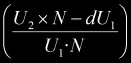Plane-Plane IntersectionGiven two planesand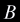, the Plücker coordinate for their intersection is given by: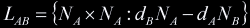Ray-Polygon and Ray-Convex Volume IntersectionThe feature of Plücker coordinates which attracted me most was the simplicity of testing ray-polygon intersections . If all the edges of a polygon are converted to their Plücker representations (taking care to order the vertices in the same direction) then taking the permuted inner product of the ray with each edge we can determine whether or not the ray passes through the polygon. For the ray to pass through the polygon, all the products must agree in sign (or one product, max two, must be zero, and the others must agree.) In the following diagram the edges move counterclockwise around the intersecting ray.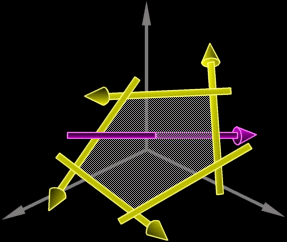We can go further to compute the point of intersection with a triangle or a quadrilateral, if an intersection did occur. Consider a triangle with edges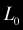,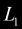,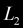, where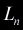is the edge opposite to the nth vertex. Take the ray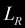and its products with edges,,, being,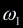,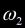respectively. If their signs agree, we know that an intersection occurred. It turns out that these are the unnormalized barycentric coordinates for the point of intersection. If we take the normalization factor to be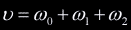then the normalized barycentric coordinates are: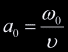,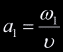,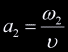.Now the point of intersection in Cartesian coordinates is the linear combination of the vertices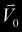,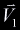,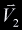. The point of intersection,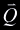, is then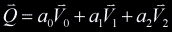.The same method can be extended for testing for intersections of rays and convex volumes (e.g. a cube). The ray needs to intersect at least one face of the volume in order to intersect with the volume. The ray-polygon test can be optimized, however, because each edge is shared. Only one permuted inner product is therefore necessary per face.

 ConclusionSadly, it's time to say goodbye. If you've made it this far without excessive convulsion, I salute you. If you've found you Zen, I salute you too! If you've found my Zen, please tell it to come home immediately. Let me know what you thought of my ramblings.- Lionel Brits (iam@cadvision.com)

 Greets and ThanksConor Stokes - for explaining a lot of conceptsPer Vognsen - for explaining what Conor meantGrandpa Rogers - for super happy fun times with the hose#flipcode and muh homies - for keeping me awake

 ReferencesArticle Series:Plücker Coordinates for the Rest of Us - Part 1Plücker Coordinates for the Rest of Us - Part 2Plücker Coordinates for the Rest of Us - Part 3Plücker Coordinates for the Rest of Us - Part 4 - Applications